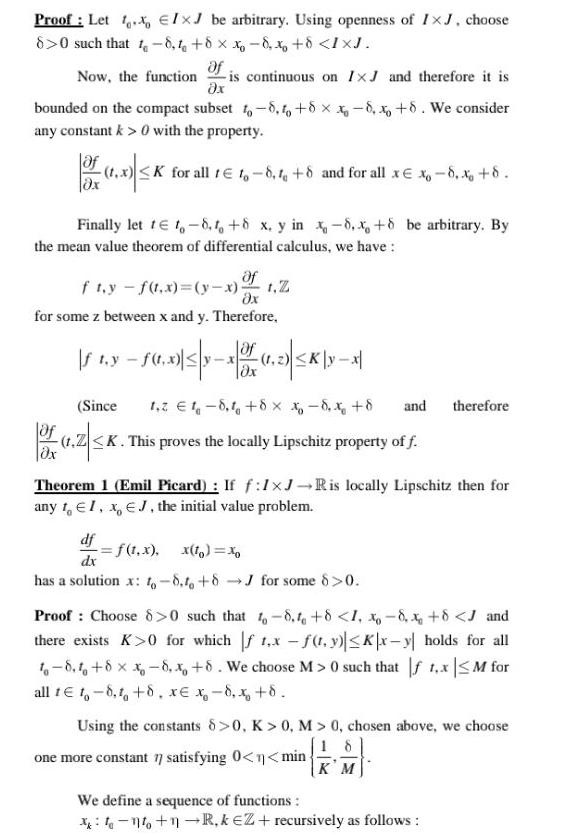Question:

# Proof Let IxJ be arbitrary Using openness of IxJ choose 8 0

Last updated: 9/18/2023Proof Let IxJ be arbitrary Using openness of IxJ choose 8 0 such that 1 8 4 8 x 8 x 8 IXJ Now the function is continuous on IxJ and therefore it is x bounded on the compact subset 10 8 0 8 x8 x 8 We consider any constant k 0 with the property of 1 3 K K for all t 1 8 8 and for all x x 8 x 8 Finally let 1 1 8 1 8 x y in 8 x 8 be arbitrary By the mean value theorem of differential calculus we have Of ft y f t x y x x for some z between x and y Therefore of fty f0 x 5 x 10 5K yx 1 2 K Ox Since 1 2 1 8 1 8 x 8 x 8 of 1 2z K K This proves the locally Lipschitz property of f Theorem 1 Emil Picard If f 1xJ R is locally Lipschitz then for any 1 EI x EJ the initial value problem df f 1 x x to xo dx has a solution x 6 8 16 8J for some 8 0 and therefore Proof Choose 8 0 such that 1 8 1 8 1 8 x 8 J and there exists K 0 for which f1 x f t y Kx y holds for all 1 8 8 x 8 x 8 We choose M 0 such that f 1 x M for all te t 8 8 xe x 8 x 8 Using the constants 8 0 K 0 M 0 chosen above we choose one more constant 77 satisfying 0 n min We define a sequence of functions KM no 1 R kEZ recursively as follows